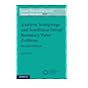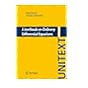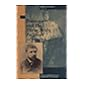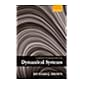Normal view MARC view ISBD view

# A textbook on ordinary differential equations / Shair Ahmad, Antonio Ambrosetti.

Material type:TextSeries: Unitext: 73.Publisher: Cham ; New York : Springer Verlag, Copyright date: ©2014Description: xiv, 304 pages : E 44.99 illustrations ; 23 cm.Content type: text Media type: unmediated Carrier type: volumeISBN: 9783319021287 (pbk.); 3319021281 (alk. paper).Genre/Form: Problems and exercises.
Contents:
1. First order linear differential equations -- 2. Theory of first order differential equations -- 3. First order nonlinear differential equations -- 4. Existence and uniqueness for systems and higher order equations -- 5. Second order equations -- 6. Higher order linear equations -- 7. Systems of first order equations -- 8. Qualitative analysis of 2 x 2 systems and nonlinear second order equations -- 9. Sturm Liouville eigenvalue theory -- 10. Solutions by infinite series and Bessel functions -- 11. Laplace transform -- 12. Stability theory -- 13. Boundary value problems -- Appendix.
Abstract: The book is a primer of the theory of ordinary differential equations. The presentation is simple, yet thorough and rigorous. Each chapter ends with a broad set of exercises that range from routine to the more challenging and thought provoking. Solutions to selected exercises can be found at the end of the book. The book contains many interesting examples on topics such as electric circuits, the pendulum equation, the logistic equation, the Lotka-Volterra system, the Laplace transform, etc, which introduce students to some interesting aspects of the theory and applications. The work is mainly intended for students of mathematics, physics, engineering, statistics, computer sciences and other areas of the sciences and social sciences that use ordinary differential equations, with knowledge of calculus and a minimal understanding of the basic concepts in linear algebra. Included are also a few more advanced topics, such as stability theory and boundary value problems, which may be suitable for more advanced undergraduate or first year graduate students. -- Source other than Library of Congress.
List(s) this item appears in: 2016-10-31
Item type Current location Call number Status Date due Barcode Item holds
Book Chennai Mathematical Institute
General Stacks
515.352 AHM (Browse shelf) Available 9983
Total holds: 0
##### Browsing Chennai Mathematical Institute Shelves , Shelving location: General Stacks Close shelf browser515.35 TAI Analytic semigroups and semilinear initial boundary value problems / 515.35 TAY Introduction to differential equations / 515.352 AGA An introduction to ordinary differential equations / 515.352 AHM A textbook on ordinary differential equations / 515.352 BAR Poincaré and the three body problem / 515.352 BAR Poincaré and the three body problem / 515.352 BRO A modern introduction to dynamical systems /

Includes bibliographical references (pages 301-302) and index.

1. First order linear differential equations -- 2. Theory of first order differential equations -- 3. First order nonlinear differential equations -- 4. Existence and uniqueness for systems and higher order equations -- 5. Second order equations -- 6. Higher order linear equations -- 7. Systems of first order equations -- 8. Qualitative analysis of 2 x 2 systems and nonlinear second order equations -- 9. Sturm Liouville eigenvalue theory -- 10. Solutions by infinite series and Bessel functions -- 11. Laplace transform -- 12. Stability theory -- 13. Boundary value problems -- Appendix.

The book is a primer of the theory of ordinary differential equations. The presentation is simple, yet thorough and rigorous. Each chapter ends with a broad set of exercises that range from routine to the more challenging and thought provoking. Solutions to selected exercises can be found at the end of the book. The book contains many interesting examples on topics such as electric circuits, the pendulum equation, the logistic equation, the Lotka-Volterra system, the Laplace transform, etc, which introduce students to some interesting aspects of the theory and applications. The work is mainly intended for students of mathematics, physics, engineering, statistics, computer sciences and other areas of the sciences and social sciences that use ordinary differential equations, with knowledge of calculus and a minimal understanding of the basic concepts in linear algebra. Included are also a few more advanced topics, such as stability theory and boundary value problems, which may be suitable for more advanced undergraduate or first year graduate students. -- Source other than Library of Congress.